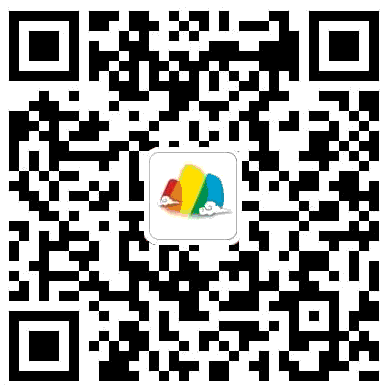# 小学数学应用题

2018-12-18 09:16:00

1429

解答应用题，既要综合应用小学数学中的概念性质、法则、公式、数量关系和解题方法等最基本的知识，还要具有分析、综合、判断、推理的能力。这也是为什么孩子觉得难的原因，今天小编整理了小学阶段典型的50道常考应用题，希望大家能认真做，熟练掌握每种题型，对期末的考试也会有很大的帮助哦！

1

288÷（10-1=32（元）

32×10=320（元）

2

3箱苹果重45千克。一箱梨比一箱苹果多5千克，3箱梨重多少千克？

3

4

5

6

3.5-4.5-?3.5=3.5-1=2.5（千米）

2.5÷（4.5-3.5=2.5÷1=2.5（小时）

7

32.5×2+5）÷（4+1=65+5）÷5=70÷5=14（吨）

14×4-5=56-5=51（吨）

8

400-10×4）÷（4+5=400-40）÷9=360÷9=40（米）

40×2+10=80+10=90（米）

9

455-30×6）÷（6+5=455-180）÷11=275÷11=25（元）

25+30=55（元）

10

11

12

13

1500+1000）÷（1500-1000=2500÷500=5（天）

1500×（5-1=1500×4=6000（千克）

14

0.45÷（8-5=0.45÷3=0.15（元）

8个练习本比8支铅笔贵的钱数：

0.15×8=1.2（元）

3.8-1.2）÷（5+8=2.6÷13=0.2（元）

15

360÷[10×6÷（8-6]=360÷[10×6÷2]=360÷30=12（辆）

360÷[10×6÷（8-6+10]=360÷[30+10]=360÷40=9（辆）

16

720×3-1200）÷80=960÷80=12（天）

720+80)×12+1200=800×12+1200=9600+1200=10800(米）

17

2×（12÷3=2×48（个）

1800÷（8+4=18000÷12=150（双）

150×2÷3=100（双）

18

120÷（30×2-40=120÷20=6（天）

30×6=180（袋）

180×2=360（袋）

19

90÷（4×5+10=3（元）

3×4=12（元）

20

572÷（10+1=52

52×10=520

21

22

23

24

“小红给小华5本，两人故事书的本数就相等”这一条件，可知小红比小华多（5×2）本书，用共有的36本去掉小红比小华多的本数，剩下的本数正好是小华本数的2倍。

36-5×2）÷2=13（本）

13+5×2=23（本）

25

5桶油重量相等，如果从每只桶里取出15千克，则5只桶里所剩下油的重量正好等于原来2桶油的重量。原来每桶油重多少千克？

26

27

35+17=52（人）

52+35=87（人）

28

29

8×2=16（千米）

30

21+20+19）÷2=30（个）

31

18-5×2=8（米）

32

33

34

35

2张桌子和5把椅子的价钱相等”这一条件，可以推出4张桌子就相当于10把椅子的价钱，买4张桌子和6把椅子共用640元，也就相当于买16把椅子共用640元。

640÷16=40（元）

40×5÷2=10O（元）

36

5年前父亲的年龄是（45-5）岁，儿子的年龄是（45-5）÷4岁，再加上5就是今年儿子的年龄。

37

“如果从甲桶倒入乙桶18千克，两桶油就一样重”可推出：甲桶油的重量比乙桶多（18×2）千克，又知“甲桶油重是乙桶油重的4倍”，可知（18×2）千克正好是乙桶油重量的（4-1）倍。

12×4=48（千克）

38

20-2-1=17（题）

39

“从两车头相遇到两车尾相离”，两车所行的路程是两车身长之和，即（240+264）米，速度之和为（20+16）米。根据路程、速度和时间的关系，就可求得所需时间。

40

41

50×12=600（米）

42

43

“只把宽增加2厘米，面积就增加12平方厘米”，可求出原来的长是：（12÷2）厘米，同理原来的宽就是（8÷2）厘米，求出长和宽，就能求出原来的面积。

44

45

15×2=30（千米）

46

8×4+5×4+12=64（个）

8×4×2=64（个）

47

1路和2路下次同时发车时，所经过的时间必须既是12分的倍数，又是18分的倍数。也就是它们的最小公倍数。

6+36=636

48

15-3=12（年）

49

60-1=59（支）

50